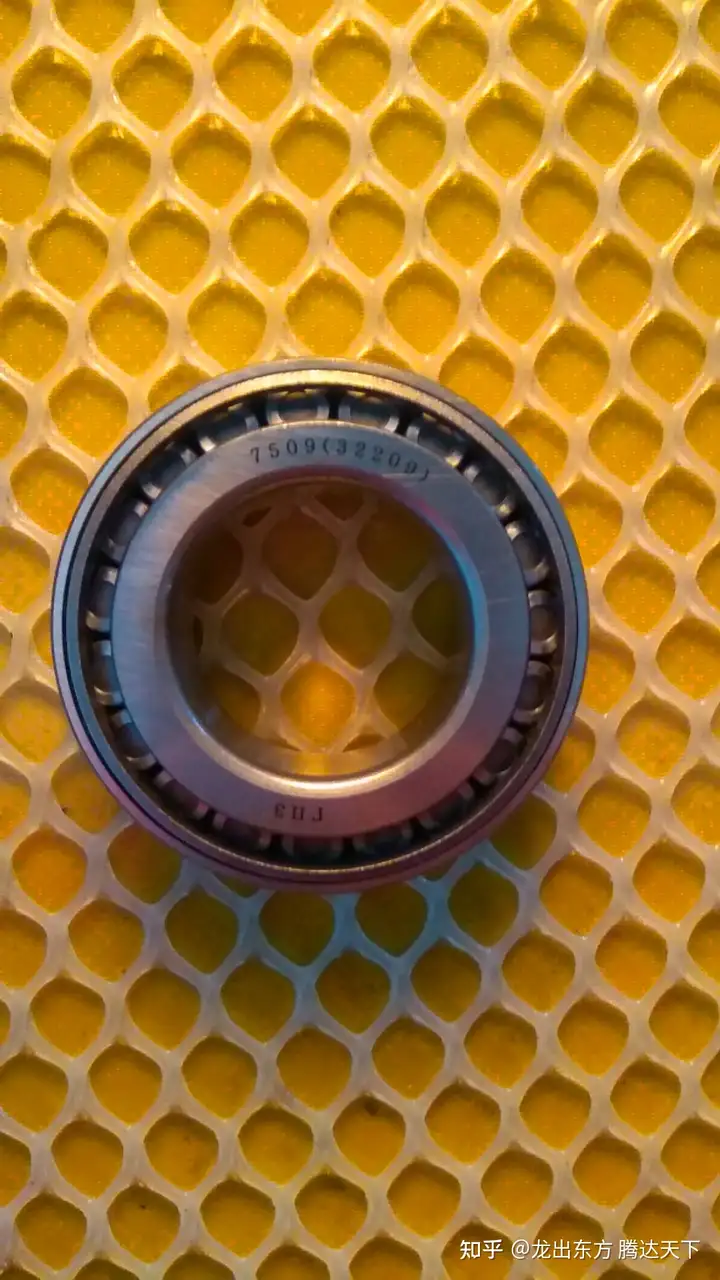## 轴承知识-推力球轴承常识简介（平面推力轴承使用方法）（运转世界大国龙腾 龙出东方腾达天下 高端三类调心滚子轴承 龙腾轴承厂 刘兴邦 ）

kN

A － 较小负荷常数，见轴承尺寸表

n － 轴承转速，r/min

α= 90° P = Fa

α≠ 90° P = XFr + YFa

Fa/Fr＞e X = 0.66 Y =1 e = 1.25

Fa/Fr＞e X = 0.92 Y =1 e = 2.17

Fa/Fr＞e X = 0.66 Y = 1 e = 1.25

Fa/Fr≤e X = 1.18 Y = 0.59 e = 1.25

Fa/Fr＞e X = 0.92 Y = 1 e = 1.25

Fa/Fr≤e X = 1.9 Y = 0.55 e = 2.17

α= 90° P0 = Fa

α≠ 90° P0 = Fa + 2.3Fr·tanα

Fa － 径向负荷，N

Fr － 轴向负荷，N

α － 接触角

X — 径向负荷系数

Y — 轴向负荷系数

## 猜你喜欢